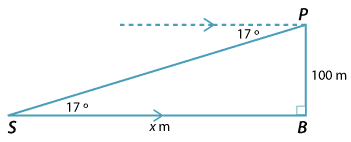#### Example 4

From the top of a cliff, 100 m above sea level, the angle of depression to a ship sailing past is 17°. How far is the ship from the base of the cliff, to the nearest metre?

#### Solution

With problems like these, always draw a diagram. The diagram shows the top of the cliff $$P$$, the ship $$S$$ and the base of the cliff $$B$$.  Let $$SB = x$$ m be the distance of the ship from the cliff.

By alternate angles, $$\angle PSB$$ = 17°.

Hence

\begin{align}\text{tan} \ 17° \ &= \frac{100}{x} \\ x \ &= \ \frac{100} {\text{tan} \ 17°} \\ &= \ 327.0852\ldots\ \text{m}\end{align}

The distance is 327 m (to the nearest metre).Detailed description